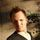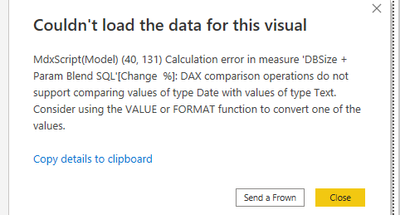cancel
Showing results for
Did you mean:## Conditional Formatting on Matrix Row level based on formula

Hi,

Need a bit of dax help from the wizards.

I'm trying to create a colour scale where the colour of a cell in a matrix table depends on the % increase/decrease from the previous value (in row).

Please note this is in Customer > Database level

So in the below table, Customer 1, DB1 would show a 200% increase from 2022-05 (10) to 2022-06 (30)

 customer database 2022-05 2022-06 2022-07 Customer 1 DB1 10 30 20 DB2 30 50 100 Customer 2 DB1 5 15 5 DB2 10 10 10

The colour scale should be something along the lines of:

<-25% decrease : Colour 1

>-25% - 0% : Colour 2

>0%: Colour 3

Hope thats enough to make sense!

1 ACCEPTED SOLUTIONFrequent Visitor

This is an interesting one that I had to play around with a little. Anyone who can optimize this solution, please do.

Basically you create a measure in DAX to calculate the percentage between this month and last month first, then you base your conditional formatting on a field like so:Here is the DAX measure I made to do this:

``````Change  % =

VAR cumulativeMinusOne = CALCULATE( SUM('Table'[Value]), PARALLELPERIOD('Calendar'[Date],-1,Month), 'Calendar'[Date] <= MAX('Table'[Year-Month]))

VAR cumulativeMinusTwo = CALCULATE( SUM('Table'[Value]), PARALLELPERIOD('Calendar'[Date],-2,Month), 'Calendar'[Date] <= MAX('Table'[Year-Month]))

VAR valueLastMonth = cumulativeMinusOne - cumulativeMinusTwo
VAR valueThisMonth = SUM('Table'[Value])
VAR changePercentage = DIVIDE( valueThisMonth, valueLastMonth, BLANK() )

RETURN IF( DIVIDE( valueThisMonth, valueLastMonth, BLANK()) <> BLANK(),  DIVIDE( valueThisMonth, valueLastMonth, BLANK()) -1, BLANK() )``````
6 REPLIES 6Frequent Visitor

Hi all,

As I suspected, the above example is far more complex than it needs to be. Please see this link for the usage of the function DATEADD(). Far more comprehensive.

You can quite easily replace the first three variables with this one function, that is meant for exactly this purpose.

MartijnFrequent Visitor

This is an interesting one that I had to play around with a little. Anyone who can optimize this solution, please do.

Basically you create a measure in DAX to calculate the percentage between this month and last month first, then you base your conditional formatting on a field like so:Here is the DAX measure I made to do this:

``````Change  % =

VAR cumulativeMinusOne = CALCULATE( SUM('Table'[Value]), PARALLELPERIOD('Calendar'[Date],-1,Month), 'Calendar'[Date] <= MAX('Table'[Year-Month]))

VAR cumulativeMinusTwo = CALCULATE( SUM('Table'[Value]), PARALLELPERIOD('Calendar'[Date],-2,Month), 'Calendar'[Date] <= MAX('Table'[Year-Month]))

VAR valueLastMonth = cumulativeMinusOne - cumulativeMinusTwo
VAR valueThisMonth = SUM('Table'[Value])
VAR changePercentage = DIVIDE( valueThisMonth, valueLastMonth, BLANK() )

RETURN IF( DIVIDE( valueThisMonth, valueLastMonth, BLANK()) <> BLANK(),  DIVIDE( valueThisMonth, valueLastMonth, BLANK()) -1, BLANK() )``````Hi, thanks for the comprehensive response! I feel like this could work...just need a bit of help with the fields

i have...

table[value]

calendar[date] - from date dimension

however i dont have year-month in the table, only in the date dimension. I only have a date field in the table. Will we need to adjust?

edit: ok i created year-month in the table however i got the following response when creating the conditional formattingFrequent Visitor

You need to make sure that the year-month column is of type Date.

Make a connection between this year-month column and calendar[date].this worked, thank you, thank you, thank you!Frequent Visitor

Martijn